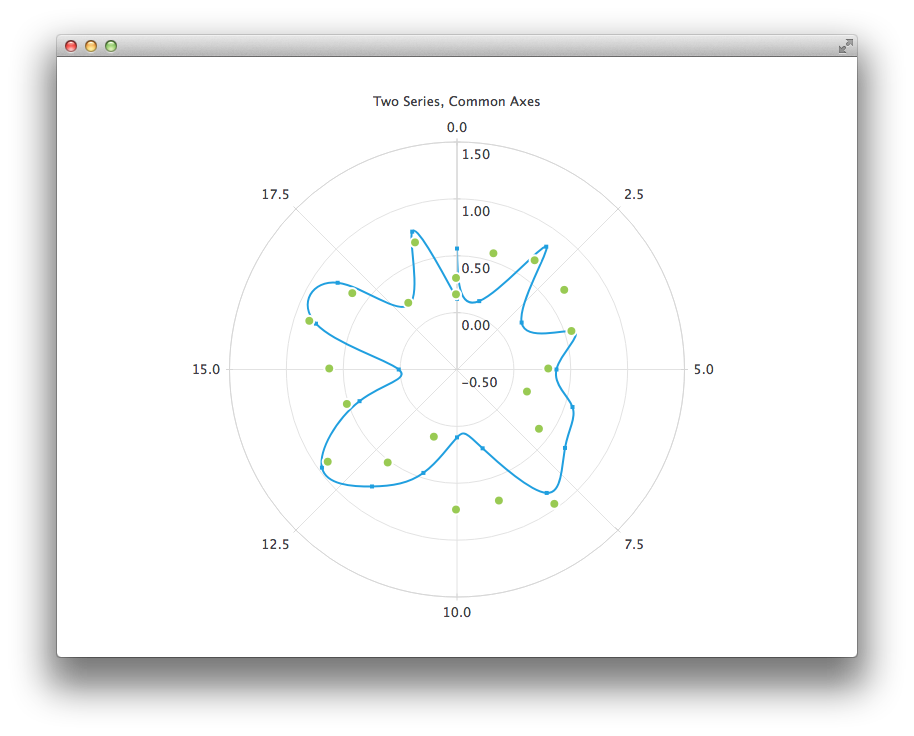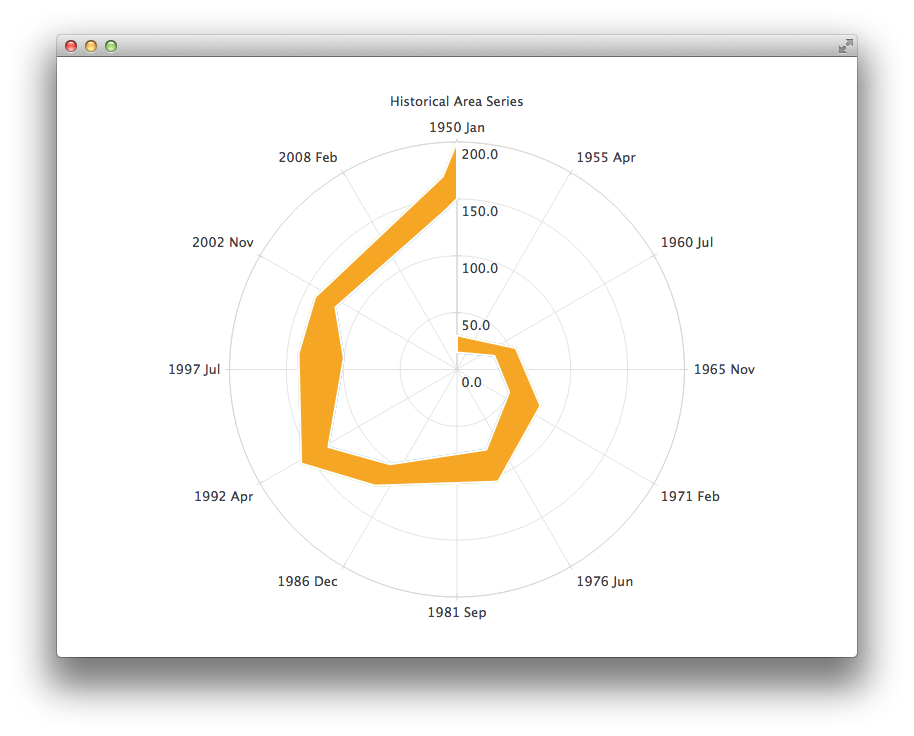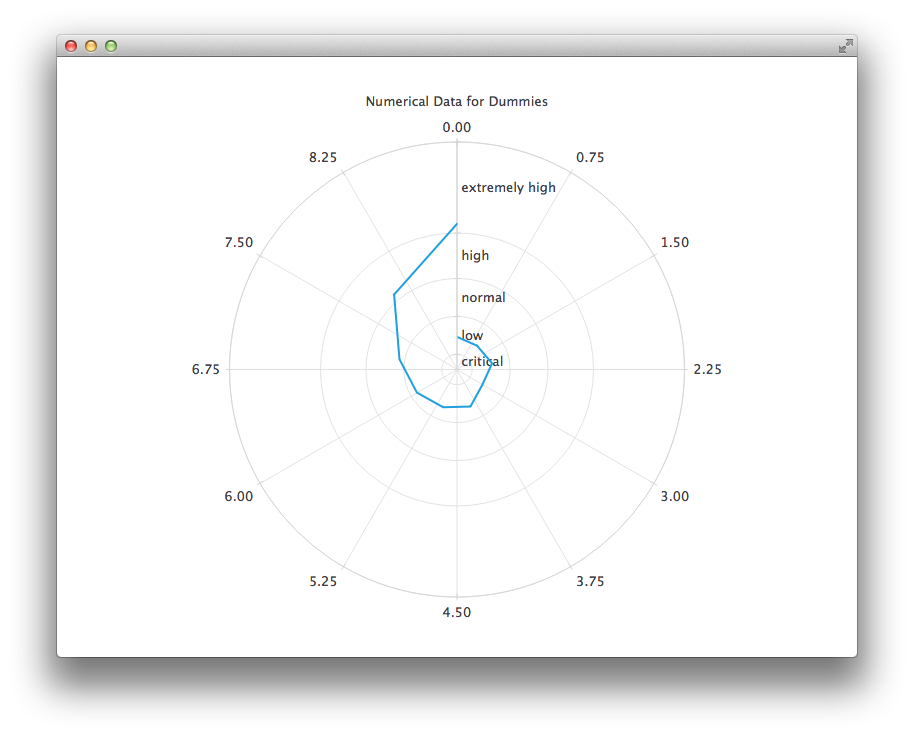# Qml Polar Chart

This is a demonstration on how to use a polar chart in your QML application.

## Running the Example

To run the example from Qt Creator, open the Welcome mode and select the example from Examples. For more information, visit Building and Running an Example.

## Using Polar Charts in Qt Quick Applications

We begin with a chart that has a spline series and a scatter series with random data. Both series use the same axes.```PolarChartView {
title: "Two Series, Common Axes"
anchors.fill: parent
legend.visible: false
antialiasing: true

ValueAxis {
id: axisAngular
min: 0
max: 20
tickCount: 9
}

ValueAxis {
min: -0.5
max: 1.5
}

SplineSeries {
id: series1
axisAngular: axisAngular
pointsVisible: true
}

ScatterSeries {
id: series2
axisAngular: axisAngular
markerSize: 10
}
}

// Add data dynamically to the series
Component.onCompleted: {
for (var i = 0; i <= 20; i++) {
series1.append(i, Math.random());
series2.append(i, Math.random());
}
}```

The next example shows a chart with some accurate historical data for which we need to use a DateTimeAxis and a AreaSeries.```PolarChartView {
title: "Historical Area Series"
anchors.fill: parent
legend.visible: false
antialiasing: true

DateTimeAxis {
id: axis1
format: "yyyy MMM"
tickCount: 13
}
ValueAxis {
id: axis2
}
LineSeries {
id: lowerLine
axisAngular: axis1

// Please note that month in JavaScript months are zero based, so 2 means March
XYPoint { x: toMsecsSinceEpoch(new Date(1950, 0, 1)); y: 15 }
XYPoint { x: toMsecsSinceEpoch(new Date(1962, 4, 1)); y: 35 }
XYPoint { x: toMsecsSinceEpoch(new Date(1970, 0, 1)); y: 50 }
XYPoint { x: toMsecsSinceEpoch(new Date(1978, 2, 1)); y: 75 }
XYPoint { x: toMsecsSinceEpoch(new Date(1987, 11, 1)); y: 102 }
XYPoint { x: toMsecsSinceEpoch(new Date(1992, 1, 1)); y: 132 }
XYPoint { x: toMsecsSinceEpoch(new Date(1998, 7, 1)); y: 100 }
XYPoint { x: toMsecsSinceEpoch(new Date(2002, 4, 1)); y: 120 }
XYPoint { x: toMsecsSinceEpoch(new Date(2012, 8, 1)); y: 140 }
XYPoint { x: toMsecsSinceEpoch(new Date(2013, 5, 1)); y: 150 }
}
LineSeries {
id: upperLine
axisAngular: axis1

// Please note that month in JavaScript months are zero based, so 2 means March
XYPoint { x: toMsecsSinceEpoch(new Date(1950, 0, 1)); y: 30 }
XYPoint { x: toMsecsSinceEpoch(new Date(1962, 4, 1)); y: 55 }
XYPoint { x: toMsecsSinceEpoch(new Date(1970, 0, 1)); y: 80 }
XYPoint { x: toMsecsSinceEpoch(new Date(1978, 2, 1)); y: 105 }
XYPoint { x: toMsecsSinceEpoch(new Date(1987, 11, 1)); y: 125 }
XYPoint { x: toMsecsSinceEpoch(new Date(1992, 1, 1)); y: 160 }
XYPoint { x: toMsecsSinceEpoch(new Date(1998, 7, 1)); y: 140 }
XYPoint { x: toMsecsSinceEpoch(new Date(2002, 4, 1)); y: 140 }
XYPoint { x: toMsecsSinceEpoch(new Date(2012, 8, 1)); y: 170 }
XYPoint { x: toMsecsSinceEpoch(new Date(2013, 5, 1)); y: 200 }
}
AreaSeries {
axisAngular: axis1
lowerSeries: lowerLine
upperSeries: upperLine
}
}
// DateTimeAxis is based on QDateTimes so we must convert our JavaScript dates to
// milliseconds since epoch to make them match the DateTimeAxis values
function toMsecsSinceEpoch(date) {
var msecs = date.getTime();
return msecs;
}```

And the final example with a chart that uses a CategoryAxis to make the data easier to understand.```PolarChartView {
title: "Numerical Data for Dummies"
anchors.fill: parent
legend.visible: false
antialiasing: true

LineSeries {
min: 0
max: 30
CategoryRange {
label: "critical"
endValue: 2
}
CategoryRange {
label: "low"
endValue: 7
}
CategoryRange {
label: "normal"
endValue: 12
}
CategoryRange {
label: "high"
endValue: 18
}
CategoryRange {
label: "extremely high"
endValue: 30
}
}

axisAngular: ValueAxis {
tickCount: 13
}

XYPoint { x: 0; y: 4.3 }
XYPoint { x: 1; y: 4.1 }
XYPoint { x: 2; y: 4.7 }
XYPoint { x: 3; y: 3.9 }
XYPoint { x: 4; y: 5.2 }
XYPoint { x: 5; y: 5.3 }
XYPoint { x: 6; y: 6.1 }
XYPoint { x: 7; y: 7.7 }
XYPoint { x: 8; y: 12.9 }
XYPoint { x: 9; y: 19.2 }
}
}```

Files: